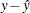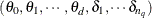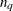# The TPSPLINE Procedure

### OUTPUT Statement

OUTPUT OUT=SAS-data-set <keyword …  keyword> ;

The OUTPUT statement creates a new SAS data set that contains diagnostic measures calculated after fitting the model.

All the variables in the original data set are included in the new data set, along with variables created by specifying keywords in the OUTPUT statement. These new variables contain the values of a variety of statistics and diagnostic measures that are calculated for each observation in the data set. If no keyword is present, the data set contains only the original data set and predicted values.

Details about the specifications in the OUTPUT statement are as follows.

OUT=SAS-data-set

specifies the name of the new data set to contain the diagnostic measures. This specification is required.

keyword

specifies the statistics to include in the output data set. The names of the new variables that contain the statistics are formed by using a prefix of one or more characters to identify the statistic, followed by an underscore (_), followed by the dependent variable name.

For example, suppose that you have two dependent variables—say, y1 and y2—and you specify the keywords PRED, ADIAG, and UCLM. The output SAS data set will contain the following variables:

• P_y1 and P_y2

• ADIAG_y1 and ADIAG_y2

• UCLM_y1 and UCLM_y2

The keywords and the statistics they represent are as follows:

RESID | R

residual values, calculated as fitted values subtracted from the observed response values:. The default prefix is R_.

PRED

predicted values. The default prefix is P_.

STD

standard error of the mean predicted value. The default prefix is STD_.

UCLM

upper limit of the Bayesian confidence interval for the expected value of the dependent variables. By default, PROC TPSPLINE computes 95% confidence limits. The default prefix is UCLM_.

LCLM

lower limit of the Bayesian confidence interval for the expected value of the dependent variables. By default, PROC TPSPLINE computes 95% confidence limits. The default prefix is LCLM_.

diagonal element of the hat matrix associated with the observation. The default prefix is ADIAG_.
coefficients arranged in the order of, whereis the number of unique data points. This option can be used only when there is only one dependent variable in the model. The default prefix is COEF_.